TRIGONOMETRY

Areas of focus:

One revolution is 360o, and is also 2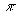radians. Thus, due to linear proportionality of the two scales, the conversion from x degrees to y radians is: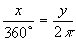One radian is equal to 3.14159..., and is normally approximated by 3.14. The following table gives equivalent angles in degrees, radians, and revolutions.

 Degrees Radians Revolutions 0o 0 0 30o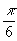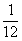45o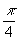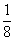60o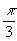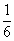90o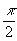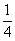180o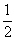270o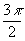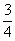360o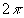1

The trigonometric functions are named sine, cosine, tangent, cotangent, secant, and cosecant. A trigonometric function has one argument that is an angle and will be denoted "". In writing the trigonometric functions one uses the abbreviated forms: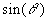,,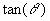,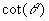,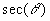, and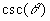, respectively. Also, sometimes these are written as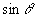,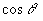,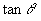,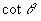,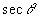, and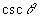, respectively.

The value of each trigonometric function for an acute angle (<90o) can be directly related to the sides of a right triangle. Consider the angle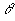in the following figure. The values of the trigonometric functions for this angle are given as: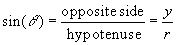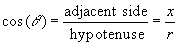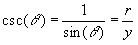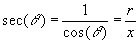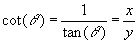Note: the exponents of trigonometric functions follow a special rule. If the exponent "n" is positive, then one writes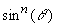in place of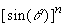. For example,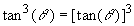The same rule does not apply to negative exponents since the exponent "-1" is reserved for the inverse trigonometric function.

In this figure,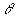and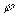are complementary angles, meaning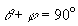. Examination of the basic relation between the trigonometric functions and the sides of the triangle reveal the following relations between the complementary anglesand.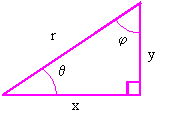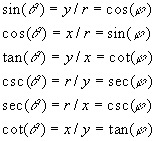Since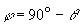, we can also write:The Pythagorean theorem states that for a right triangle, as shown, there exists a relation between the length of the sides given be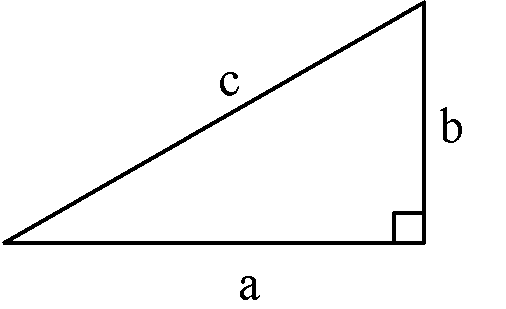a2 + b2 = c2

There are also Pythagorean triples for (a,b,c), such as (3,4,5), (5,12,13) and (7,24,25) sided triangles, and all constant multiples of these triplets (e.g., (6,8,10)).

From the Pythagorean Theorem of plane geometry we know that x2 + y2 = r2. This can be used to derive a basic relation between the sine and cosine functions.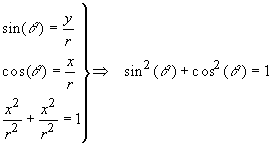GO TO PART 2